# Introductory Data Science using R

Lecture 1: The Data Science Framework

## Structure

• Lecture 1: The data science framework
• Lecture 2: Using R
• Lecture 3: Data science with R
• Lecture 4: Exploratory analysis of Kiva.org data

## The scientific inquiry

data + model —> understand

• Not new, arises in many fields
• Natural sciences
• Econometrics
• Psychology
• Sociology
• etc.Giuseppe Piazzi’s observations in the Monatliche Correspondenz, September 1801.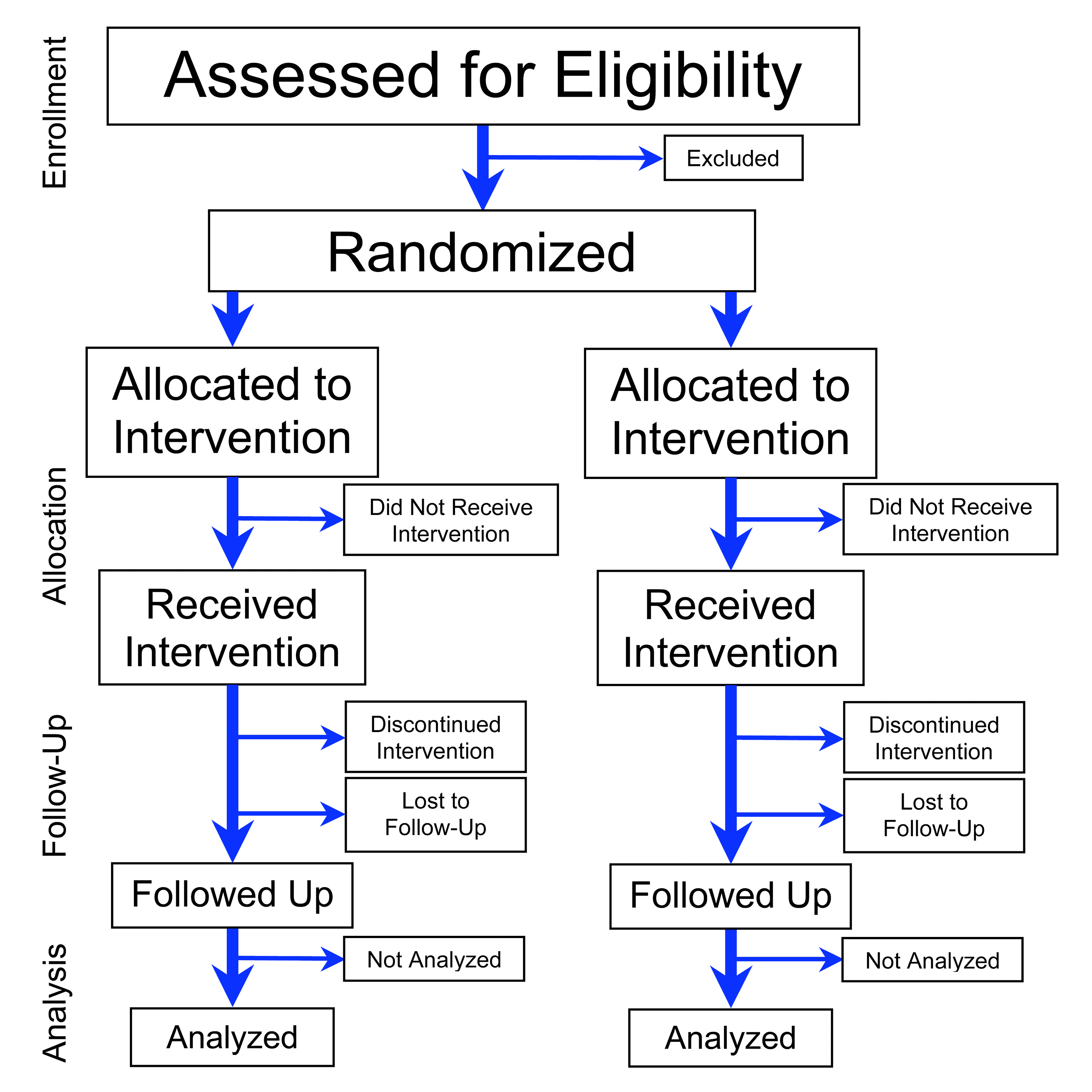• Design of experiments; randomised control trials.
• Sir Ronald Fisher (1890–1962).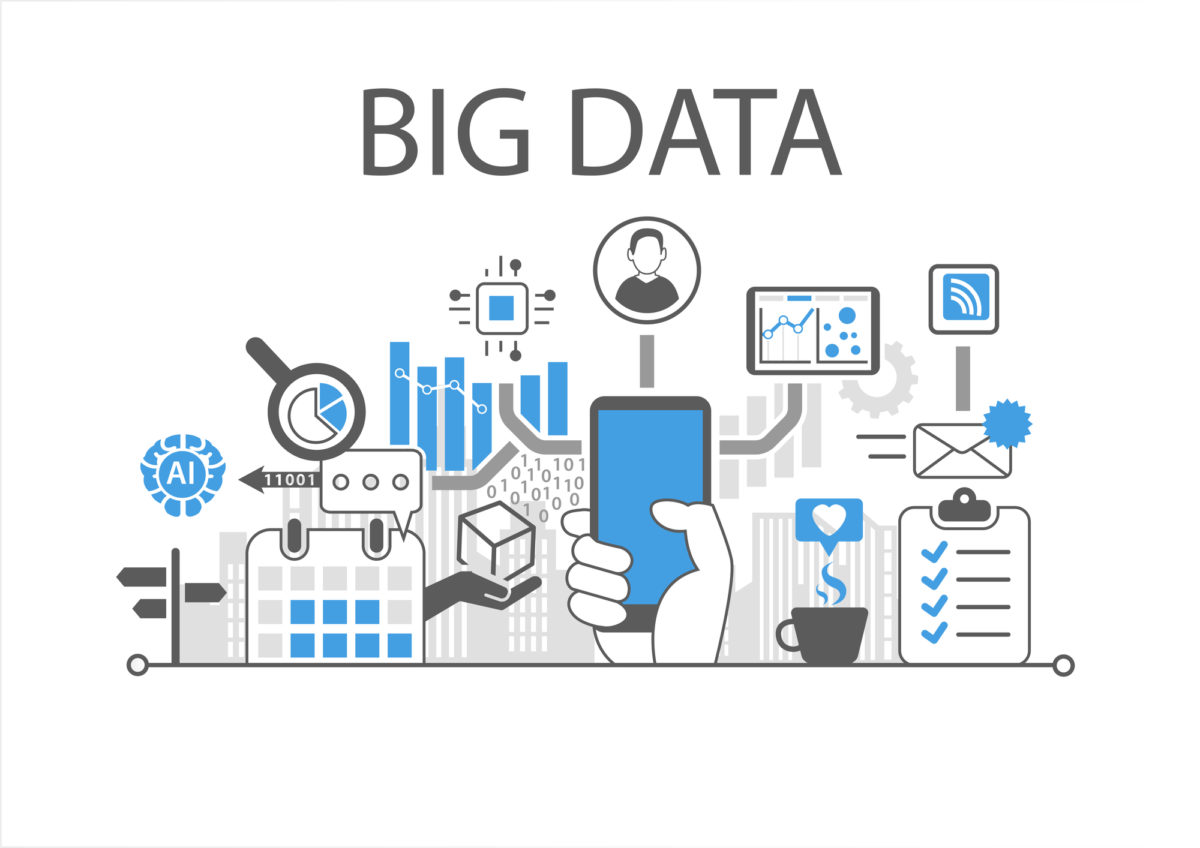Data is now available by happenstance, and not just collected by design.

## Big Data

The more we measure, the more we don’t understand

• Breadth vs depth paradox; Big p Small n; The curse of dimensionality
• Ethics; privacy

define: Data Science

The “concept to unify statistics, data analysis, machine learning and their related methods” in order to “understand and analyze actual phenomena” with data.

• Multi-displinary field
• Goal: extract knowledge and insights from structured and unstructured data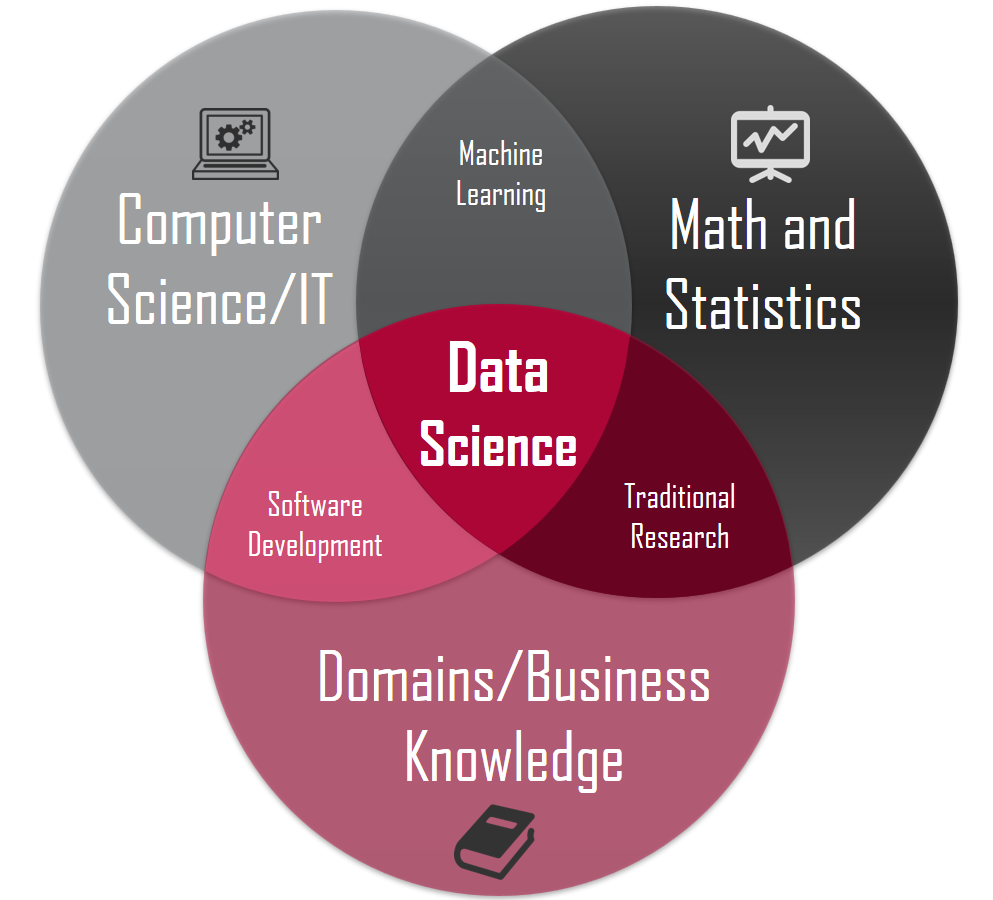### Examples of Data Science problems

Real-world problems from the Alan Turing Institute

• Real-time jammer detection, identification and localization in 3G and 4G networks
• Automated matching of businesses to government contract opportunities
• Using real-world data to advance air traffic control
• Personalised lung cancer treatment modelling using electronic health records and genomics

### Examples of Data Science problems

Real-world problems from the Alan Turing Institute

• Identify potential drivers of engaging in extremism
• News feed analysis to help understand global instability
• Improved strength training using smart gym equipment data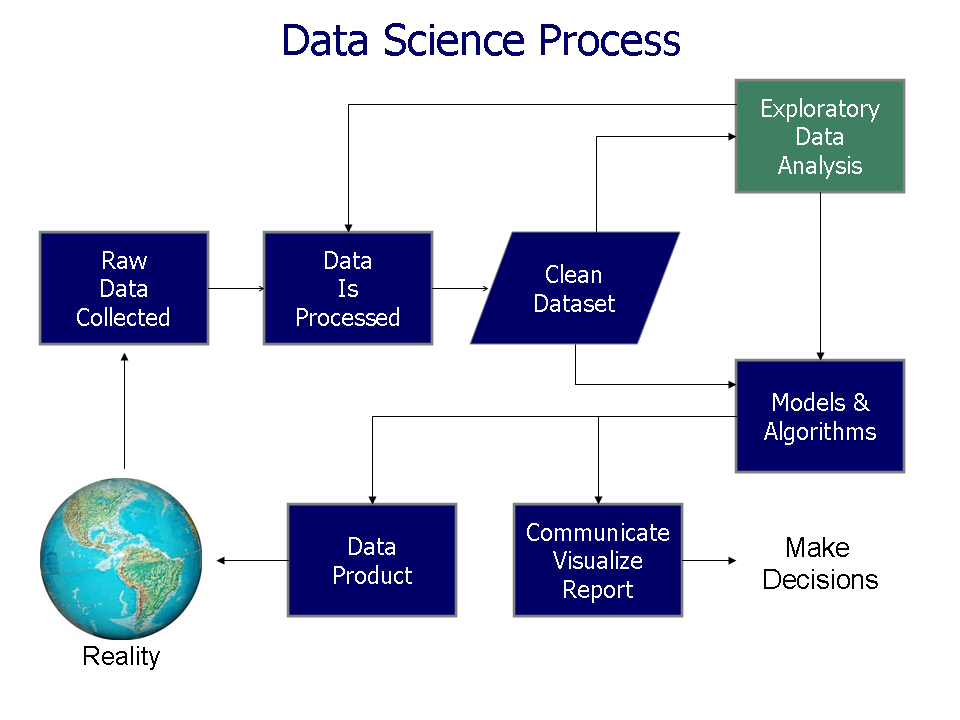## Scope: Exploratory

• Focus on transform and visualise
• Modelling requires a specific skill set (Stats or ML)
• GOAL: Generate many promising leads that you can later explore in more depth

### Machine Learning vs Statistics

Statistics aims to turn humans into robots.

• Concept of “statistical proof”
• Often interest is inference

Machine learning aims to turn robots into humans.

• Make sense of patterns from big data
• Often interest is prediction

There’s a sea of data, but most of it is undrinkableData neglect: data cleaning is tedious and complex

### 80-20 rule of Data Science

• Most time is spent cleaning up data
• Affectionally called data “wrangling”
• [TBA] Data Readiness levels (Bands A, B and C)

### Types of data

1. Structured data
• Data is in a nicely organised repository
• E.g. Tables, matrices, etc.
2. Unstructured data
• Information does not have a predefined data model
• E.g. images, colours, text, sound, etc.

### Types of data

1. Continuous data
• Measurements are taken on a continuous scale e.g. height, weight, temperature, GDP, distance, etc.
• Usually arises from physical experiments
2. Discrete data
• Measurements which can only take certain values e.g. sex, survey responses (Likert scales), occupation, ratings, ranks, etc.
• Usually arises in social sciences

### Types of data

TreatmentContinuous DataCategorical Data
Import classnumericfactor, ordinal
VisualiseHistograms, density plots, scatter plot, box & whisker plot, pie chartsBar plots,
Summarise5-point summariesFrequency tables

### Exploratory Data Analysis

3. Use what you learn to refine your questions and/or generate new questions.

More on this later…

### Modelling

$$y_i = \alpha + \beta x_i + \epsilon_i$$ $$\epsilon_i \sim \text{N}(0,\sigma^2)$$

• EDA does not help in providing statistical proof, nor give predictions
• To do this, engage in statistical or ML models
• Many types of models, depending on what question you want answered

### The R programming language

R is a language and environment for statistical computing and graphics https://www.r-project.org/about.html

• It is free and open source
• Runs everywhere
• Supports extensions
• Engaging community

## Kiva.org data set

https://www.kaggle.com/kiva/data-science-for-good-kiva-crowdfunding#kiva_loans.csv

## Exercise

• What exploratory analyses would you conduct on this data set?
• What other data do you need to supplement your analyses?
• What questions do you aim to answer?

Questions?

# Supplementary material

## Inference vs Prediction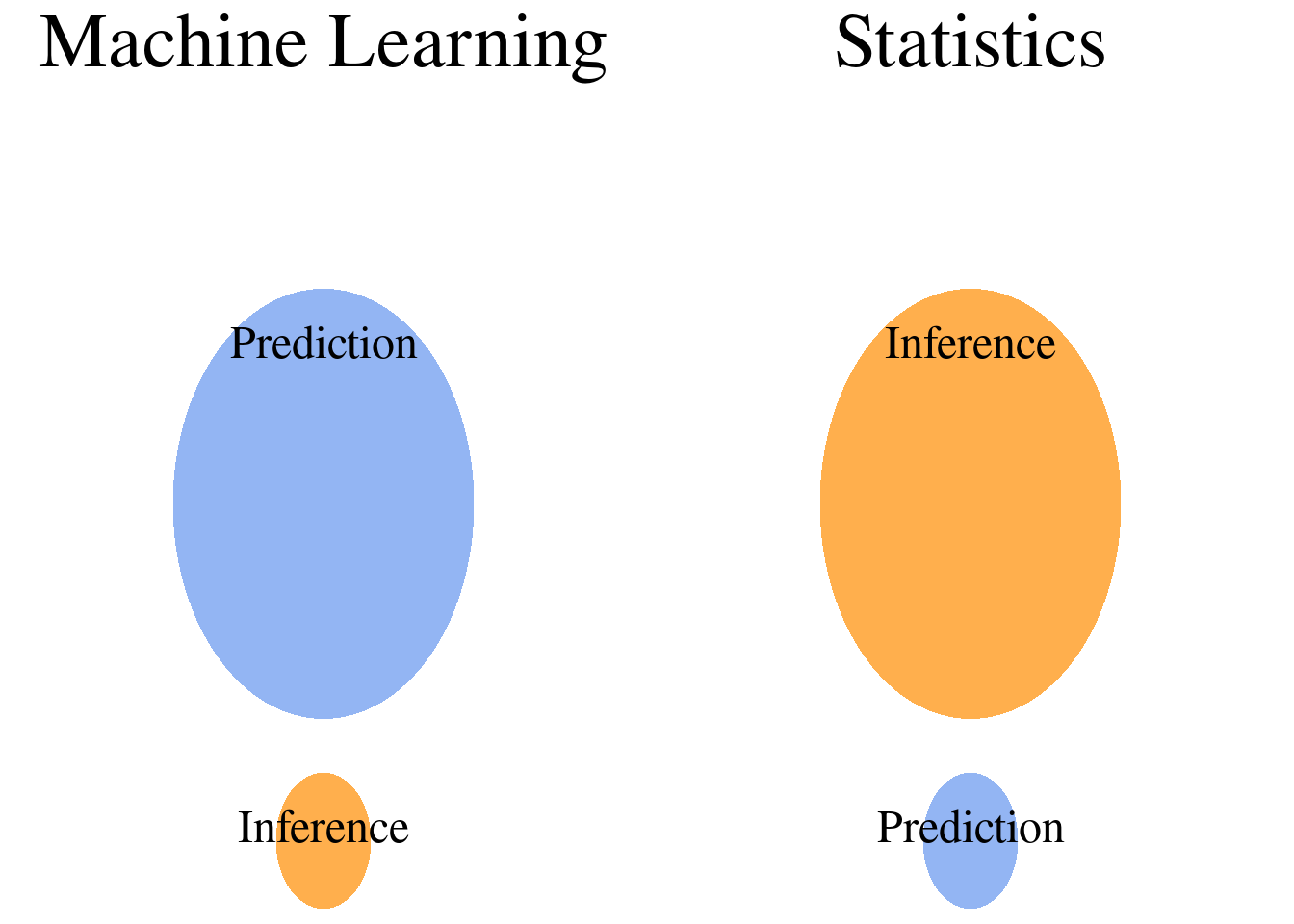Source: datascienceblog.com

## Model interpretability

• Model interpretability is necessary for inference
• In a nutshell, a model is interpretable if we can “see” how the model generates its estimates
• c.f. Blackboxes
• Interpretable models often uses simplified assumptions

## Model complexity

• A complex model is often better at prediction tasks
• “More parameters to tune”
• However, model interpretability suffers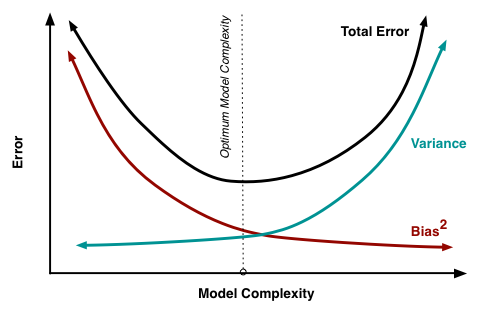$$E[f(x) - \hat f (x)]^2 = \text{Bias}^2[\hat f(x)] + \text{Var}[\hat f(x)] + \sigma^2$$

### Linear regression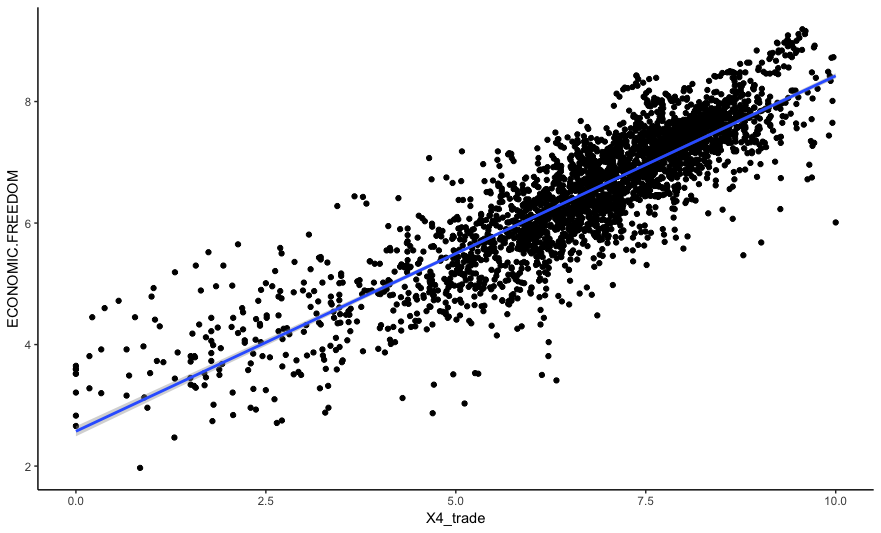Economic freedom = 2.6 + 0.6 Trade

### Neural networks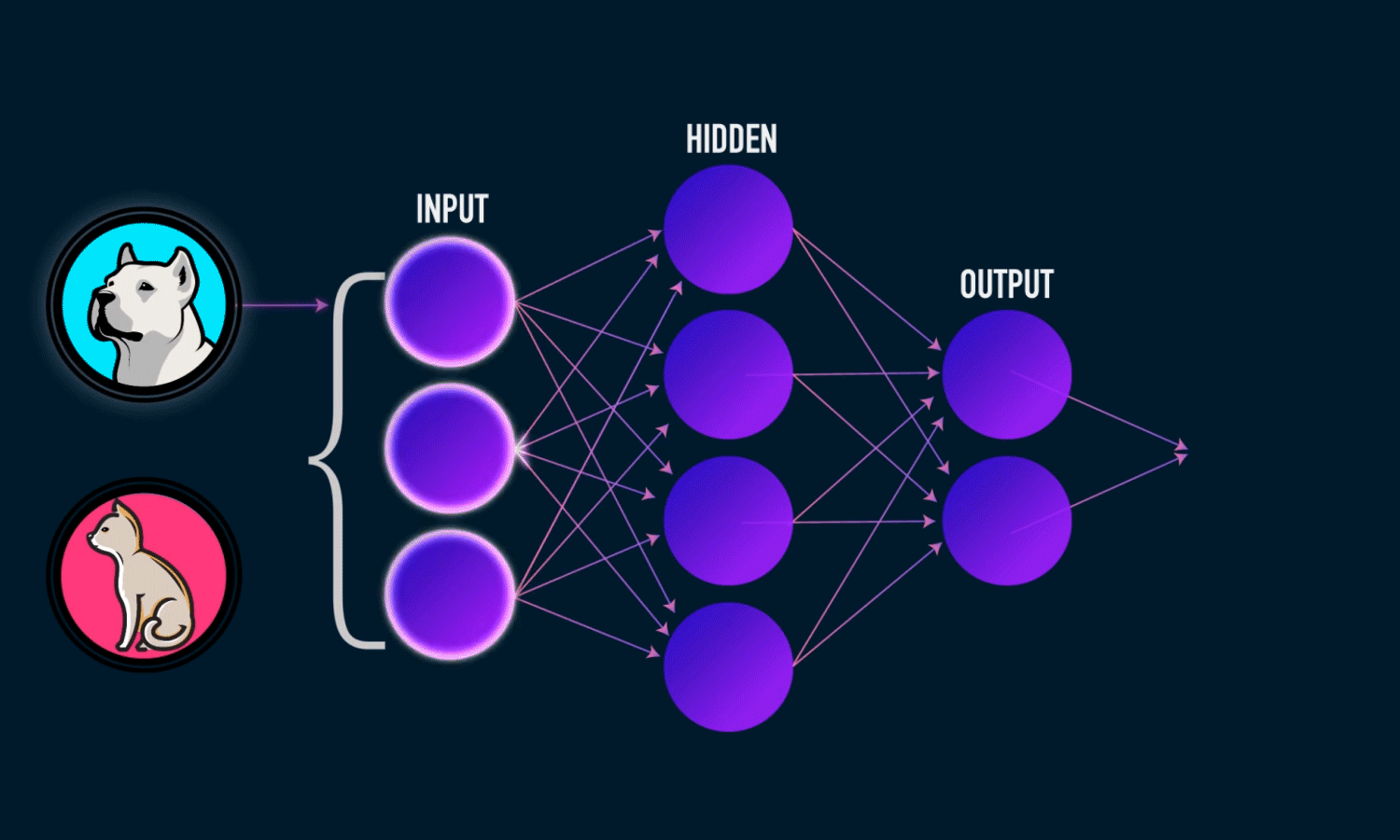Source: towardsdatascience.com

### Survey Methodology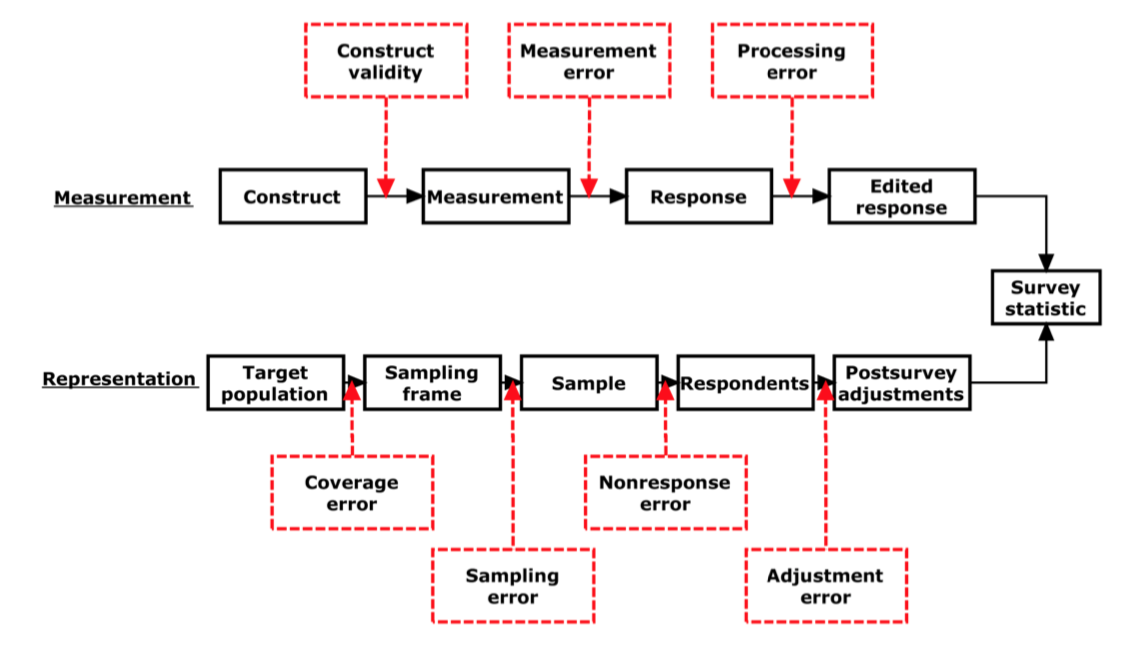Source: Groves et al. (2009)

### Three populations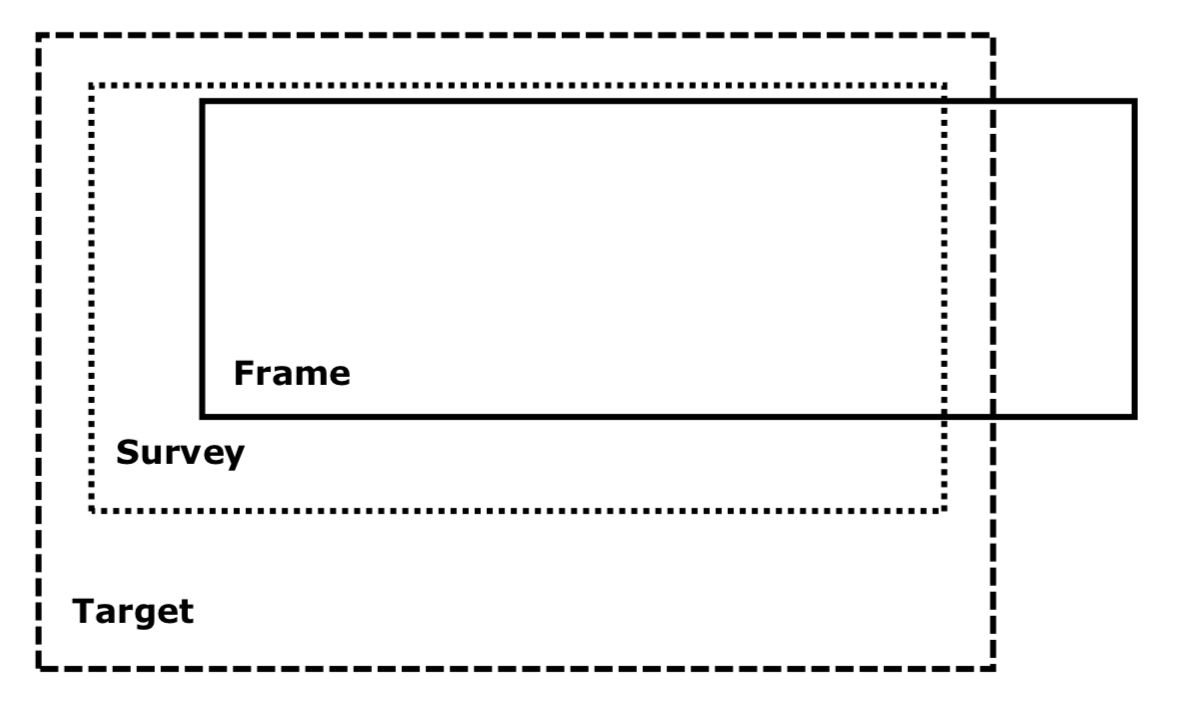### Sampling design for BSA survey

• Target: Adults aged 18 or over in GB
• Survey: Private households south of the Caledonian Canal

Multistage design:

• Stratify by postcode sectors
• Simple random sampling of addresses
• Simple random sampling of individuals

From 60mil people, obtained 3,297 respondents in final sample.